# Assessment of the finite element solutions for 3D spontaneous rupture using GeoFEM

## Abstract

Numerical simulations of spontaneous shear rupture on a planar fault using a slip-weakening model in a three-dimensional uniform elastic medium were conducted using a parallel FE code, GeoFEM, which was originally developed for the solid-Earth simulations on the Earth Simulator. The aim was to evaluate the accuracy and applicability of GeoFEM to earthquake rupture. The present numerical results are compared with published results obtained using a finite-difference (FD) method and a boundary integral (BI) method for rupture times and time functions of slip, slip rate, and shear stress at two particular points on the fault plane. The effects of mesh size and damping on the attenuation of spurious oscillations were also examined in a range of simulations. The appropriate degree of damping depends on mesh size and must be introduced in order to obtain reliable numerical solutions; weak damping leads to significant oscillation and strong damping to artificially low rupture speeds and low slip rates. Our results indicate that mesh size should be sufficiently small to allow the inclusion of a few grids in the cohesive zone, as shown in other numerical methods. The solutions by GeoFEM that have the appropriate mesh size and damping are quite similar to those obtained using the FD and BI methods, the difference between them being generally less than 2% in terms of the rupture time and 5.2% in terms of the peak slip rate.

## 1. Introduction

Understanding spontaneous rupture is of fundamental importance for understanding the mechanisms involved in earthquakes, and must be investigated numerically because it cannot be solved analytically. FD and BI methods have often been used for the numerical simulation of spontaneous rupture, and the validity and accuracy of these methods as a function of grid size in numerical computations has been extensively studied, for example, by Day et al. (2005) and Dalguer and Day (2006, 2007). At the same time, an alternative and sophisticated simulation method has been developed using a finite element (FE) method, and this has also been used for earthquake rupture problems, as referred to by, for example, Oglesby and Day (2001), Oglesby and Archuleta (2003), Ma et al. (2008), and Barall (2009). The FE method, established using the variational principle, assembles the whole system from individual elements in which the basic shape functions are used to integrate the field variables. Integration in space over the nodes that belong to the different elements is not therefore necessary, and the restriction of using structured grids in FD no longer applies. The FE method is more flexible when dealing with complicated geometries by using an unstructured mesh. Such a feature is attractive in real simulations of rupture that involve complicated fault geometries and heterogeneous materials. Although the FE method has been developed to a considerable degree, and significant achievements have been seen in the fields of analysis of solids and structures, it is important to compare the accuracy of simulations by the FE method with those by the FD and BI methods for simulations of spontaneous rupture (Moczo et al., 2007; Galis et al., 2009, 2010).

We herein describe our numerical simulation of spontaneous shear rupture on a planar fault in a three-dimensional (3D) uniform elastic medium using the FE method. Using the same problem as that of Day et al. (2005), we attempt to evaluate the accuracy of the FE method and quantitatively compare this with that obtained by the FD and BI methods. This problem was originally defined for the SCEC (Southern California Earthquake Center/U.S. Geological Survey) Dynamic Earthquake Rupture Code Verification Exercise (Harris et al., 2009).

We used a parallel FE code, GeoFEM, which was originally developed for the solid-Earth simulations on the Earth Simulator (Iizuka et al., 2000), and has been applied to quasi-static viscoelastic deformation (e.g. Hyodo and Hirahara, 2004). The original GeoFEM was capable of large-scale static structural simulations with a constant frictional contact using a master-slave method. In its use of a master-slave method, our approach is different from the split-node technique (Andrews, 1999) that has been used in fault modeling. In our study, we decided to add some new features to the existing GeoFEM scheme in order to solve dynamic rupture problems. Firstly, we introduced a slip-weakening friction law in order to model the variation in friction that occurs during fault rupture, by which we could vary the friction as a linear function of slip, rather than using a constant value. Secondly, because fault rupture is a nonlinear dynamic problem, a modified version of the conventional Newmark time integration scheme was adopted to ensure the proper conversion of energy and momentum in time for nonlinear dynamic analysis. After giving details of the FE formulation, we present an extensive comparison between the accuracy obtained in our FE simulations and that obtained in the benchmark results of the FD and BI models as reported by Day et al. (2005). Finally, we also describe the results of our investigations into the dependency of the FE results on grid size and damping parameters.

## 2. Finite Element Formulations

In the following section, we describe the details of our FE formulations. We address the problem of contact using a slip-weakening friction law, we use an energy-momentum-conserving time integration method for dynamic nonlinear analysis, and we select damping parameters that reduce the oscillation of high-frequency modes.

### 2.1 Contact element with friction

In simulating dynamic fault motions, a split-node technique (Andrews, 1999; Day et al., 2005; Ma et al., 2008) has, to date, enjoyed widespread use for modeling the boundary conditions of faults in both FD and FE calculations. However, in general, contact problems are usually modeled by means of a master-slave type of contact element, which does not require the complete overlapping of nodes at the fault, and can be applied to more general unstructured and non-conforming mesh discretization on the fault plane. A node-to-segment contact element without friction was previously used in the original version of GeoFEM. The detailed formulation of the frictionless contact and the method by which contact constraint is enforced through the use of a penalty can be found in Iizuka et al. (2000). We herein give the derivation of the condition of consistent stiffness of the contact element using the slip-weakening friction law proposed by Ida (1972). We assume a simple linear slip weakening and a friction coefficient μ expressed as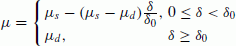(1)

where the subscripts s and d denote static and dynamic friction, respectively, δ is the slip across the fault and δ0 is the critical slip distance. A frictional contact problem that followed a Coulomb plastic law was described by Peric and Owen (1992), in which the frictional coefficient was constant. In the slip-weakening friction law of Eq. (1), the frictional coefficient μ is no longer constant. Instead, it is a linear function of the slip δ, until 8 reaches a value δ0, above which the coefficient μ assumes a constant value μ d . Therefore, the derivation of the tangent stiffness representing the traction-displacement relation must be rewritten.

At a certain time step T + ΔT, the contact force on the surface can be written as:(2)

where T+ΔTf t and T + ΔTf n are the frictional and normal force, respectively, T+ΔTt and T+ΔTn are the unit vectors in the direction of the slip and the contact surface normal, respectively. The displacement difference u at a contact point can be expressed explicitly in terms of the nodal displacements u i on both sides of the contact surface (Appendix A). By differentiating Eq. (2) with respect to u, we obtain the tangential contact stiffness matrix at time T + ΔT :(3)

where the symbol denotes the outer product of two vectors, and we assume that the contact surfaces do not deform very much, so that the differential of the contact normal TTn with respect to du can be ignored. In the first term on the right-hand side of Eq. (3), the term d(μ|T+ΔTf t |)/du can be further separated into two terms:(4)

As shown in Fig. 1, the slip on the contact surface δ can be written as(5)

where T+ΔTu t is the slip vector on the contact point between the two sides of the contact surface at time T + ΔT, after relative slip initially occurs, but not a frictional path dependent slip. Therefore, from Eq. (1), dμ/du in the second term of the right-hand side of Eq. (4) can be expressed as(6)

where T+ΔTt′ denotes the unit vector in the direction of the slip increment (T+ΔTu t Tu t )/|TTu t Tu t |, as shown in Fig. 1. The first term on the right-hand side of Eq. (4) can be written as:(7)

where T+ΔTD n = p n (T+ΔTn T+ΔTn), and p n is the penalty in the contact surface normal. A very large penalty is applied in the contact normal direction in order to limit the penetration of the slave node into the master segment. This is one of the most commonly-adopted methods for introducing constrained conditions into variational equations. Theoretically, the non-penetration condition on the contact surface requires an infinitely large penalty. However, too large a penalty would lead to an ill-conditioned matrix and cause numerical problems in the solution. Herein, the value of the penalty in the contact normal direction is chosen to be 1010 times the Young’s modulus of the material, a value that is sufficiently large to limit the penetration within a scale of displacement ×10−10 but does not lead to the occurrence of an ill-conditioned stiffness matrix. The same value of penalty is also used for the contact tangent direction p t . Since the direction of slip varies continuously, it is necessary to derive the derivative of T+ΔTt with respect to u:(8)

whereis the trial friction force assuming that no slip occurs at time T+ΔT and I is a 3 × 3 unit matrix. Figure 2 gives an explanation of the return mapping procedure used to update the frictional force. When the value ofexceeds that of the friction force, it is ‘pulled back’ to a frictional force that follows the slip-weakening frictional law. T+ΔTD t is the derivative ofwith respect to u and can be written as(9)

By combining Eqs. (4), (6), (7) and (8), Eq. (3) finally reduces to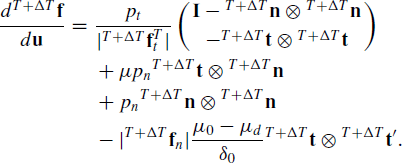(10)

The last term on the right-hand side of Eq. (10) denotes the contribution from the slip-weakening friction law, without which a simple frictional contact formulation with a constant frictional coefficient is obtained.

### 2.2 Dynamic time integration scheme

A particular case of the Newmark method is the trapezoidal rule scheme, which may be used as one of the implicit time integration methods, and is also popular and unconditionally stable for linear dynamic analysis (Zienkiewicz and Taylor, 2002). However, when being applied to nonlinear dynamic problems, such as the dynamic fault rupture discussed herein, the trapezoidal rule scheme becomes rather unstable. Such instability comes from the uncontrolled oscillation of the sum of the potential energy and the momentum. The trapezoidal rule scheme does not guarantee the conservation of energy-momentum throughout the duration of the model run. In order to overcome these adverse characteristics, a generalized energy method, originally proposed by Chung and Hulbert (1993), and systematically reviewed by Kuhl and Crisfield (1999), was adopted and implemented in our own code. In simple terms, the generalized energy method modifies the Newmark method by applying the equation of motion to a general mid-point instead of to the end point. The internal force is then calculated using the displacements at the mid-point. Such a modification leads to a time integration that is based on a low numerical dissipation at lower frequencies and a high numerical dissipation at higher ones, so that the oscillation of the total energy is suppressed. Further details of this method can be found in Appendix B.

### 2.3 Use of damping

In the dynamic analysis of a system that has many degrees of freedom, it is the high-frequency modes that are the sources of the numerical instability. In simulations of fault rupture, the high-frequency modes can be damped using numerical damping methods. The simple approach of Rayleigh damping is used in many finite-element analysis programs, such as ABAQUS (2006) and NASTRAN (2005). In these programs, reliable results for numerically sensitive structural systems may be obtained by the increased damping of the higher-frequency modes of the system, but this approach suffers from a lack of physical justification and significant errors may result. A damping term that is proportional to the velocity is introduced into the equation of motion (see Eq. (B.1) in Appendix B). In Rayleigh damping, the damping matrix C is expressed as a linear combination of the mass and stiffness matrices in the form(11)

where C, M and K are the damping, mass and stiffness matrices, respectively, while α M and α K are proportional coefficients of mass and stiffness. Herein, we do not restrict our approach to the use of the global matrices of Eq. (11); instead, we assign different values of α M and α K to individual elements. The damping ratio, which is a dimensionless measure that describes how much the oscillations in a system decay after a disturbance (Bathe, 1996), can be expressed as a function of angular frequency ω(ω = f/2π) or in terms of a frequency f, using α M and α K in the case of Rayleigh damping:(12)

It is well known that under mass-proportional damping, a greater degree of damping is applied to the low-frequency modes of the system, and that under stiffness-proportional damping, the damping is more effective at the higher-frequency modes. Because high-frequency artificial oscillations should be reduced in our simulations of fault rupture, only stiffness-proportional damping is applied here. The damping matrix may then be written as(13)

where the subscript K of α is omitted for simplicity. Then, Eq. (12) may be reduced to:(14)

Because of the absence of the mass-proportional coefficient, the damping ratio ξ is a linear function of ω (or f). For example, if the frequency mode f = 100 Hz needs to be reduced by 50%, then, by using Eq. (14), the corresponding damping coefficient can be calculated as α = ξ/(πf) = 0.5/(100π) ≈ 1.592 × 10−3. This value of α leads to a damping ratio ξ = 2.5% at a frequency mode of f = 5 Hz. It is noteworthy that Semblat (1997) investigated a rheological interpretation of Rayleigh damping and found an approximate relationship between the quality factor Q and the damping ratio as 1/ Q ≈ 2ξ for weak to moderate Rayleigh damping. In addition, it is also worth mentioning that a similar artificial viscosity damping was introduced in a series of FD simulations by, for instance, Day et al. (2005), Dalguer and Day (2007) and Rojas et al. (2009). Such a damping is treated as a device to suppress short-wavelength oscillations. Therefore, it is to regularize the numerical solution, rather than to represent a physical damping, just as in the case of Rayleigh damping.

In all our simulations of rupture, we aimed to achieve optimal damping such that the high-frequency modes that generate spurious oscillations were reduced, while, at the same time, minimizing any unfavorable damping effects that might reduce any physically-meaningful high-frequency motion. To this end, we assigned different values of stiffness-proportional damping coefficients to different positions. Further details of this technique are given in the following section.

## 3. Fault Model Description

### 3.1 Geometry of fault

Our numerical simulations were conducted for a planar fault embedded in a 3D infinite isotropic elastic space. The test fault geometry and material properties were the same as those used by Day et al. (2005). The fault was placed on the xy-plane (Fig. 3(a)), and the rupture area was confined to a rectangular area of 30 km by 15 km (Fig. 3(b)). The model space of 60 km × 40 km × 40 km was much larger than that of the fault plane so that any effects caused by the reflection of waves from the model boundaries would be small. In the central part of the fault there was a square nucleation area of 3 km by 3 km in which the rupture was initiated (Fig. 3(b)). The size of this assumed nucleation zone was comparable to the theoretical critical crack size for spontaneous rupture as discussed by Day et al. (2005). It was assumed that the media on either side of the fault were elastic and homogeneous with the fundamental material properties of density ρ = 2670 kg/m3, P wave velocity V p = 6000 m/s, and S wave velocity, assuming a Poisson ratio of v = 0.25. The values of Young’s modulus and shear modulus can be derived from the above values, all of which are listed in Table 1. A small amount of damping was introduced to suppress spurious oscillations, as discussed in Section 3.3, and accordingly, the material was viscoelastic. The initial values of normal and shear stress (σ n and τ0) applied to the fault are shown in Table 2. The shear stress initially applied in the nucleation area was slightly higher than the static yielding stress so that the rupture could initiate spontaneously. The rupture then propagated through the fault, following the slip-weakening law, whose parameters are also listed in Table 2, until it reached the boundary of the prescribed rupture area (30 km × 15 km). Outside this area, no rupture was allowed, the static yielding stress being set to infinity.

The fault region in which the rupture occurred was finely discretized. Outside the rupture area, the mesh size increased with distance from the rupture area in the x-, y-, and z-directions in order that the total number of elements could be minimized. An example of our fault discretization is shown in Fig. 4, where the elements that are in contact with the rupture area of the fault are 100-m cubes, and the largest element size is 1000 × 1000 × 2000 m3.We performed numerical simulations for elements of 100-m, 300-m, and 500-m cubes to assess the effect of mesh size. The total numbers of elements were 2,450,000, 320,000, and 60,000 for mesh sizes in the rupture area of 100 m, 300 m, and 500 m, respectively.

In order to obtain the initial conditions for the numerical simulation of the dynamic rupture, a quasi-static loading was first applied using the boundary conditions listed in Table 3, as also illustrated in Figs. 3(a) and 4. By fixing the nodal displacements u z on the surface of z to −20 km, and those of u y on the surfaces of y to ±20 km, we applied a uniform normal stress σ zz of 120 MPa to the surfaces of z = +20 km as well as finite controlled displacements u x on surfaces of x = ±30 km to both the lower and upper blocks but in opposite directions, in order to obtain the initial shear and normal stresses. Because a uniform normal stress was applied, the initial shear stress on the fault could be determined using the predefined distribution of initial frictional coefficients μ0 given in Table 2, in order to generate the same initial stress states as those used in the benchmark simulations. Such a quasi-static loading was necessary for the FE simulations because it guaranteed the equilibrium of forces throughout the whole model prior to the dynamic rupture.

### 3.3 Parameters for dynamic analysis

The dynamic rupture was triggered by a sudden drop in frictional coefficient from μ0 to μ s within the 3 km × 3 km nucleation area. The dynamic rupture then started to propagate in both the x- and y-directions. The time increment for dynamic analysis was chosen as 0.005 s per step. The duration of the whole simulation was set to 7.5 s because by this time the prescribed 30 km × 15 km rupture area was completely ruptured. The stiffness-proportional damping described in the previous section was assigned to the following three parts of the mesh: (1) a layer of elements on either side of the fault; (2) the outermost layer of elements on the constraint boundaries; (3) the rest of the homogeneous medium. We applied damping to Part (1) in an attempt to reduce the spurious oscillations in slip and slip rate, using three different cases of stiffness-proportional damping coefficient α = 5α0, α0 and 0.625α0, where α0 = 1/(100π) = 3.183 × 10−3 (yielding α = 1.592 × 10−2, 3.183 × 10−3 and 1.989 × 10−3), corresponding to damping ratios ξ = 5%, 1% and 0.625%, respectively, at a frequency of 1 Hz from Eq. (14). For Part (2), a relatively large stiffness-proportional damping coefficient α = 100 α0 = 1/π = 0.3183 was used to reduce the amplitudes of the waves reflected from the model boundaries. A very small stiffness-proportional damping coefficient α = 0.005 α0(1.592 × 10−5) was used for Part (3), in order to minimize the effect of damping throughout the whole system given that Part (3) occupies most of the model space. Although the sudden change of damping coefficients between Parts (2) and (3) may result in some degree of non-physical reflection, such a reflection will not affect the results within the simulation duration because the outermost boundaries are far away from the rupture area.

## 4. Numerical Results

We performed numerical simulations for several cases using different mesh sizes (100 m, 300 m, and 500 m) and different stiffness-proportional damping coefficients (α = 0.625α0, α0 and 5α0 at the fault-layer elements), in order to examine the effects of these conditions on the numerical results. Among these, the case with the mesh size of 100 m and α = 0.625α0 gives the best result, and accordingly, we now discuss the result of this case (the reference case) in some detail, in order to compare it with the benchmark results of Day et al. (2005). We then compare the simulation results obtained for different mesh sizes and different α-values of the damping coefficient. The simulations were all performed on cluster machines in an MPI-based parallel style. For the finest mesh size (100 m) with 2,450,000 elements, the simulations were run in parallel on 32 processors with a total required memory of 14.6 GB. It took about 120 hours per CPU to complete the 7.5 s simulation.

### 4.1 Results in comparison with benchmarks

The fault rupture that nucleated in the central square started to propagate spontaneously on the fault, following the slip-weakening frictional law. The rupture accelerated rapidly to reach subsonic velocities of propagation both in the in-plane direction along the x-axis and in the anti-plane direction along the y-axis.

Figure 5 shows the cohesive zone of the simulated rupture along the x-axis (fault along the in-plane) and along the y-axis (fault along the anti-plane) for our reference case, compared with the results obtained by Day et al. (2005). The cohesive zone curves are plotted by selecting the time points at which the shear stress reaches the static yielding stress τ S and then drops to the dynamic frictional stress τ d at each node on the x-axis, and on the y-axis, in the rupture area. Day et al. (2005) conducted numerical simulations for many cases using a range of different methods and grid sizes. Among these, we only used the high-resolution benchmark results obtained using fine grids, including two cases of 100 m and 50 m for FD (DFM0.1 and DFM0.05) and one case of 100 m for BI (BI0.1), for comparison with our reference FE results (FE0.1). The extent of the cohesive zone generally decreases as the rupture propagates in all cases, and no significant differences can be seen (Fig. 5) in the space-time pattern of the cohesive zone between our cases and those of Day et al. (2005). A dimensionless measure of resolution N c , defined as the ratio of the width of the cohesive zone to the mesh size on the fault plane, was also compared with the benchmark results of DFM0.1 and BI0.1, given that both of these simulations used the same grid spacing (100 m) as ours. The medianand the minimumvalues of Nc obtained in our simulations were 4.6 and 3.5, respectively. Comparingandwith those in the benchmark results of DFM0.1 and BI0.1and, the differences are only 4.5% and 6%, respectively.

For the damping coefficient α in our simulation, it is found there may exist an approximating relation to the dimensionless damping parameter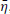, used in Day et al. (2005) and Dalguer and Day (2007), as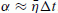, based on dimensional analysis, where Δt is the time step. Day et al. (2005) suggestedwith Δt = 0.008 s for a grid spacing of 100 m, leading to an equivalent α = 0.8 × 10−3 which was much smaller than that which we used 0.625α0 = 1.989 × 10−3. It is probably because that Day et al. (2005) applied the same level of damping globally. On the other hand, Dalguer and Day (2007) limited the damping to the fault-adjacent elements only and found the preferred higher values of the damping parameterin the range 0.2 ~ 0.4, which is close to ours.

Figure 6 shows the rupture times of our reference case superimposed on the benchmark results of Day et al. (2005). Dalguer and Day (2006) noted that the Stress-Glut method (Andrews, 1976) yielded a systematically low rupture velocity and that the Thick-Fault model (Madariaga et al., 1998) failed to match the quantitative behavior of the benchmark results. Considering these results, our distribution of rupture times is satisfactory, although slight delays in FE0.1 may be observed in both directions when the rupture fronts approach the boundaries of the 30 km × 15 km rupture area.

We now focus on the comparison of the time histories of slip, slip-rate and shear stress at two sample points within the rupture area, namely PI, which is 7.5 km away from the origin along the x-axis, and PA, which is 6.0 km away from the origin along the y-axis, as illustrated in Fig. 3(b). In Fig. 7, we compare our reference results at PI with those of Day et al. (2005). The time histories of slip and shear stress for our reference case are all very similar to those for the three benchmark cases (Fig. 7 top panel). No observable difference in rupture time is found at PI between the cases shown. For the slip rate history (Fig. 7 bottom panel), our results for the time of onset and the time of the peak slip rate show a slight delay of 0.02 s and 0.05 s, respectively, and the peak value is about 5.2% lower than the benchmark results. In the Stress-Glut method, the stress concentration is blunting (Dalguer and Day, 2006). This is caused by an underestimation of the slip in the slip-weakening friction due to the half-grid shift of particle velocity grids away from the fault. In the Thick-Fault model, additional blunting from the thick fault occurred (Dalguer and Day, 2006). In our method, however, the fault-plane stress and the fault slip are evaluated on the fault plane itself, thereby removing the possibility of this cause of rupture delay. Our method is thus more accurate than either the Thick-Fault method or the Stress-Glut method. In fact, as mentioned above, only 0.02 and 0.05 s delays in onset time are shown in Fig. 7.

Spurious oscillation occurred well after the peak slip rate in FE0.1. Figure 8 shows the time histories at PA. In general, the slip and shear stress histories of our FE reference case agree well with those of the benchmark. The time at which the shear stress reached the static yielding stress of our FE reference case was almost the same as that of the benchmark result BI0.1, and the maximum difference in rupture time in either of the other two benchmark results DFM0.1 and DFM0.05 was less than 0.03 s. The peak value of slip rate at PA in our FE result was almost the same as that of DFM0.05 and BI0.1. In contrast with the slip rate history at PI, which was relatively smooth near the peak value, the slip rate at PA clearly oscillated near the peak value. A similar oscillation may be observed in the benchmark results of DFM0.1, but not in the results of DFM0.05 or BI0.1. The cause of this difference between PI and PA may lie in the fact that the width of the cohesive zone near PA (400 m) is narrower than that near PI (500 m), where the grid size was not fine enough to capture the smoother responses, as suggested by Day et al. (2005). Moreover, they pointed out that a finer grid size is necessary for the FD method than for the BI method to obtain a smooth time history of slip rate. Analogously, it is expected that such a trend would hold for the FE method, which will be discussed in the next section.

### 4.2 Effects of mesh size and damping coefficient on FE results

In the following subsection, we discuss the dependence of the FE solution on mesh size and the stiffness-proportional damping coefficient used in the fault layer elements.

Firstly, we investigated the mesh-size dependence using three different mesh sizes of 100 m, 300 m, and 500 m. The stiffness-proportional damping coefficients for the fault layer elements for the three cases took the same value α = 5α0(= 1.592 × 10−2; Part 1 mesh, see Section 3.2). The larger damping coefficient used here is to avoid over-oscillation in coarse mesh cases of 300 m and 500 m, which will be seen in Fig. 10. Figure 9 shows the rupture times at PI and PA for different mesh sizes, indicating a longer delay of the rupture front for a coarser mesh. In addition, it may be seen that the rupture delay in the y-direction (at point PA) is longer than that in the x-direction (at point PI). This implies that the dependence on mesh is rather more obvious in the anti-plane direction than in the in-plane direction. Figure 10 shows the time histories of the slip rates at points PI and PA for the three cases considered. Although the finest mesh discretization of 100 m case gives a satisfactory result with a relatively smooth slip rate function as discussed in the preceding subsection, significant spurious oscillations were observed for the coarser meshes (300 m and 500 m). This finding suggests that stronger damping should be applied for coarser meshes in order to obtain smooth slip rate functions. In general, numerical dispersion is apparent for both the FD and FE methods (Aki and Richards, 1980), and a coarse discretization of the modeling space causes a spurious oscillation at lower frequencies. Equation (14) indicates that the damping ratio ξ decreases at lower frequencies. For computations that use a coarse mesh, stronger damping is therefore required. Day et al. (2005) reported that N c ≥ ~5 is required to obtain an accurate result using DFM. Almost the same value of Nc seems to be required for FE, because, in general, our finest mesh size (100 m) gives a satisfactory result with N c = 4.4, while coarser meshes (300 m and 500 m) with smaller values of Nc generally yield unfavorable results.

We then investigated the effect of varying the stiffness-proportional damping coefficient, by using a mesh size of 100 m with three different stiffness-proportional damping coefficients α = 5α0, α0 and 0.625α0 for the fault layer elements. Figures 11 and 12 show the simulated slip rate histories at PI and PA, respectively, using different values of α. It was found that the use of the largest value of α = 5α0 leads to a distinct delay in rupture propagation in both the x- and y-directions (see zoom-in figures on the right-hand sides of Figs. 11 and 12). There is a 0.26 s delay at point PA and a 0.17 s delay at point PI compared with the results for the smallest value of α = 0.625α0. With decreasing stiffness-proportional damping coefficients from α0 to 0.625α0, the difference is less visible at PI in Fig. 11, but is still obvious at PA in Fig. 12. In addition, the slip rate curves at PI and PA for α = 5α0 are relatively smooth, though the peak slip rates are about 10% smaller than those for α = α0 and α = 0.625α0, both of which give almost the same values of peak slip rate. It may be expected that a further decrease in α will not affect the peak slip rate very much.

From the present study, we conclude that the mesh size has a significant influence on the accuracy of our FE simulations of fault rupture. For the present fault test model, the use of a mesh size of 100 m, among our other simulation cases, yields the best accuracy. The proper selection of damping coefficient for a particular frequency can reduce spurious high-frequency oscillation while having little effect on accuracy, and can further improve the performance of the model. For the case of mesh size 100 m with α = 0.625α0, it is found that although the oscillations affect less the results of rupture times (Fig. 6) and shear traction time histories as shown Figs. 7 and 8, they do affect the accuracy of the slip rate so that it would cause difficulties for further parametric investigation. It would be helpful to adopt some smoothing algorithms (e.g., Galis et al., 2010) in our FE formulation in the future.

## 5. Conclusions

We have obtained finite element (FE) solutions of spontaneous shear rupture in a planar fault in a 3D uniform elastic medium by using a parallel FE code, GeoFEM, with some modifications for applying a slip-weakening friction law and adopting a time integration scheme that guarantees the conservation of energy and momentum. The fault model used in our study was the same as that used by Day et al. (2005) for evaluating the accuracy of a finite difference (FD) method and a boundary integral (BI) method, and permitted us to compare our results with those obtained by Day et al. (2005). Several simulations were performed in order to examine the effects of mesh size and damping parameter necessary to avoid spurious oscillations. Comparing the time histories of the slip rate and the shear stresses, and the rupture time distribution obtained in the present FE simulations with those obtained by Day et al. (2005), we found that almost the same results were obtained by the present FE method as those by the FD and BI methods, provided that the appropriate mesh size and damping coefficients were used. In the simulation of dynamic faulting with slip-weakening friction, the size of the cohesive zone must encompass more than a few grid points to express a breakdown process properly and thereby to yield reliable numerical results. The ratio of the size of the cohesive zone to mesh size for stable numerical solutions under the present FE scheme should be greater than 5, which is almost equal to that of the FD scheme and a little larger than that of BI reported by Day et al. (2005). Because the size of the cohesive zone depends on the slip-weakening parameters, the mesh size required in the numerical computations depends on these parameters. The value of the appropriate damping coefficient is determined by the mesh size, because artificial oscillations of a longer period are generated for coarser meshes. Stronger damping artificially decreases rupture velocities, peak slip rates, and the amount of slip. Through the numerical study described herein, we confirm that our FE method provides solutions to fault rupture that are almost as accurate as those given by the FD and BI methods. Spurious oscillations observed in slip rates in the present method may be further improved by introducing sophisticated smoothing algorithms as discussed in Section 4.2. The FE methods are flexible in that they may be used with an unstructured mesh discretization, and, accordingly, they are capable of simulating complicated problems even with a non-conformed mesh on a fault plane, by using a formulation that involves master-slave contact. Our study has provided standards for the required mesh and damping conditions, and confirms the accuracy of this approach for use in possible future applications.

## References

• ABAQUS 6.6, Theory manual, ABAQUS, Inc., 2006.

• Aki, K. and P. Richards, Quantitative Seismology, W. H. Freeman and company, 1980.

• Andrews, D. J., Rupture propagation with finite stress in antiplane strain, J. Geophys. Res., 81, 3575–3582, 1976.

• Andrews, D. J., Test of two methods for faulting in finite-difference calculations, Bull. Seismol. Soc. Am., 89, 931–937, 1999.

• Barall, M., A grid-doubling finite-element technique for calculating dynamic three-dimensional spontaneous rupture on an earthquake fault, Geophys. J. Int., 178, 845–859, 2009.

• Bathe, K. J., Finite Element Procedures, Prentice Hall, 1996.

• Chung, J. and G. M. Hulbert, A time integration algorithm for structural dynamics with improved numerical dissipation: the generalized—a method, J. Appl. Mech., 60, 371–375, 1993.

• Dalguer, L. A. and S. M. Day, Comparison of fault representation methods in finite difference simulations of dynamic rupture, Bull. Seismol. Soc. Am., 96, 1764–1778, 2006.

• Dalguer, L. A. and S. M. Day, Staggered-grid split-node method for spontaneous rupture simulation, J. Geophys. Res., 112, B02302, 15 pp., 2007.

• Day, S. M., L. A. Dalguer, N. Lapusta, and Y. Liu, Comparison of finite difference and boundary integral solutions to three dimensional spontaneous rupture, J. Geophys. Res., 110, B12307, doi:10.1029/2005JB003813, 2005.

• Harris, R. A., M. Barall, R. Archuleta, E. Dunham, B. Aagaard, J. P. Ampuero, H. Bhat, V. Cruz-Atienza, L. Dalguer, P. Dawson, S. Day, B. Duan, G. Ely, Y. Kaneko, Y. Kase, N. Lapusta, Y. Liu, S. Ma, D. Oglesby, K. Olsen, A. Pitarka, S. Song, and E. Templeton, The SCEC/USGS Dynamic Earthquake Rupture Code Verification Exercise, Seismol. Res. Lett., 80, 119–126, 2009.

• Hyodo, M. and K. Hirahara, GeoFEM kinematic earthquake cycle simulation in Southwest Japan, Pure. Appl. Geophys., 161, 2069–2090, 2004.

• Ida, Y., Cohesive force across the tip of a longitudinal-shear crack and Griffith’s specific surface energy, J. Geophys. Res., 77, 3796–3805, 1972.

• Iizuka, M., H. Okuda, and G. Yagawa, Nonlinear structural subsystem of GeoFEM for fault zone analysis, Pure. Appl. Geophys., 157, 2105–2124, 2000.

• Kuhl, D. and M. A. Crisfield, Energy-conserving and decaying algorithms in nonlinear structural dynamics, Int. J. Numer. Meth. Eng., 45, 569–599, 1999.

• Galis, M., P. Moczo, and J. Kristek, A 3-D hybrid finite-difference finite-element viscoelastic modelling of seismic wave motion, Geophys. J. Int., 175, 153–184, 2009.

• Galis, M., P. Moczo, J. Kristek, and M. Kristekova, An adaptive smoothing algorithm in the TSN modelling of rupture propagation with the linear slip-weakening friction law, Geophys. J. Int., 180, 418–432, 2010.

• Ma, S., S. Custodio, R. J. Archuleta, and P. Liu, Dynamic modeling of the 2004 Mw 6.0 Parkfield, California, earthquake, J. Geophys. Res., 113, B02301, 16 pp., doi:10.1029/2007JB005216, 2008.

• Madariaga, R., K. Olsen, and R. Archuleta, Modeling dynamic rupture in a 3D earthquake fault model, Bull. Seismol. Soc. Am., 88, 1182–1197, 1998.

• Moczo, P., J. Kristek, M. Galis, P. Pazak, and M. Balazovjech, The finite-difference and finite-element modeling of seismic wave propagation and earthquake motion, Acta Physica Slovaca, 57, 177–406, 2007.

• NX Nastran 4 Advanced Nonlinear Theory and Modeling Guide, UGS Corp., 2005.

• Oglesby, D. D. and R. J. Archuleta, The three-dimensional dynamics of a nonplanar thrust fault, Bull. Seismol. Soc. Am., 93, 2222–2235, 2003.

• Oglesby, D. D. and S. M. Day, Fault geometry and the dynamics of the 1999 Chi-Chi (Taiwan) earthquake, Bull. Seismol. Soc. Am., 91, 1099–1111, 2001.

• Peric, D. and R. J. Owen, Computational model for 3-D contact problems with friction based on the penalty method, Int. J. Numer. Meth. Eng., 35, 1289–1309, 1992.

• Rojas, O., E. Dunham, S. M. Day, L. A. Dalguer, and J. E. Castillo, Finite difference modeling of rupture propagation with strong velocity-weakening friction, Geophys. J. Int., 179, 1831–1858, 2009.

• Semblat, J. F., Rheological interpretation of Rayleigh damping, Jounral of Sound and Vibration, 206, 741–744, 1997.

• Zienkiewicz, O. C. and R. L. Taylor, The Finite Element Method, fifth edition, vol. 1 The basic, Butterworth Hernemann, 2002.

## Acknowledgements

The authors thank two reviewers of this article, Steven Day and Peter Moczo for valuable comments that led to an improvement of the manuscript. The computations were carried out by the parallel computer of the Earthquake and Volcano Information Center in the Earthquake Research Institute, University of Tokyo. This study was supported by the Ministry of Education, Culture, Sports, Science and Technology (MEXT) of Japan, under its Observation and Research Program for the Prediction of Earthquakes and Volcanic Eruptions.

## Author information

Authors

### Corresponding author

Correspondence to Jun Yin.

## Appendices

### Appendix A. Node-to-segment Contact Element

The displacement difference u on a virtual contact point can be expressed as a function of nodal displacements of one slave node and the corresponding master segment nodes, as follows:(A.1)

where k is the number of nodes on the master segment and N j (j = 1, 2,..., k) are the corresponding shape functions. u i is the nodal displacement vector that includes one slave node and the master segment nodes.(A.2)

where the superscripts s and m denote slave and master, respectively, and the superscript T outside the bracket denotes transposition. The slip vector on the contact surface is of the form:(A.3)

### Appendix B. Generalized Energy-momentum Method

In the Newmark method (Zienkiewicz and Taylor, 2002), the equation of motion is applied at the end-point in time T + ΔT as in Eq. (B.1), with time integrations to displacements and velocities as follows,(B.1)(B.2-1)(B.2-2)

where M, C and K are the mass, damping and stiffness matrixes and F e is an external force vector. ΔT is the time increment, and the superscript T and T + ΔT denotes the start and end of the time step. β1 and β2 are the two Newmark parameters. For the trapezoidal rule, 2β1= β2 = 0.5 was selected.

In the generalized energy method, Kuhl and Crisfield (1999), introduced a mid-point parameter α (note that the α used here is different from the stiffness-proportional coefficient in Section 2.3) with which the equation of motion is applied at time T +αΔT (see (B.3)), and the displacements, velocities, accelerations and external forces are interpolated correspondingly by (B.4)(B.3)(B.4-1)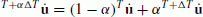(B.42)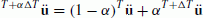(B.43)(B.44)

By substituting (B.4-1), (B.4-2) and (B.4-3) into (B.2-1) and (B.2-2), we obtain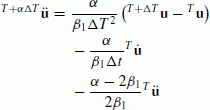(B.51)(B.52)

For a nonlinear system, the internal forces F i , which represent the forces due to deformation, depend nonlinearly on the displacements, and the equation of motion must be solved iteratively. By applying a Newton-Raphson strategy and a Taylor expansion, the force residual R may be evaluated at T + αΔT and written as:(B.6)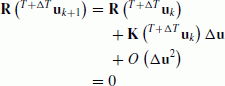(B.7)

where K is the deformation-dependent tangential stiffness and the subscript k denotes the kth iteration, () denotes functions with respect to variables . By differentiating (B.6) with respect to displacement T+ΔTu, the tangent stiffness can be derived:(B.8)

The internal force F i may then be calculated from the strains at T + αΔT, T+αΔTε and is in the form:(B.9)

## Rights and permissions